Kuang Algorithm Engineer&Data Mining Engineer

# 《Joint Extraction of Entities and Relations Based on a Novel Tagging Scheme》阅读笔记

### 2 Contributions

• 提出一种新的标注方法，将联合抽取实体和关系的任务转化为标注任务
• 使用不同的end-to-end模型来解决该问题，效果好于绝大多数现有的串行抽取和联合抽取方法
• 提出一种偏置损失函数，使用该损失函数可以使相关实体间的联系变得更强

### 3 Method

##### Tagging Scheme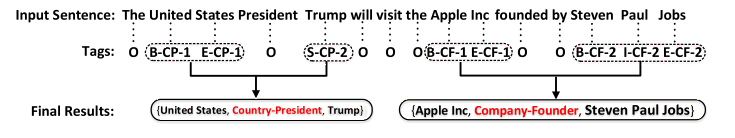##### The End-to-End Model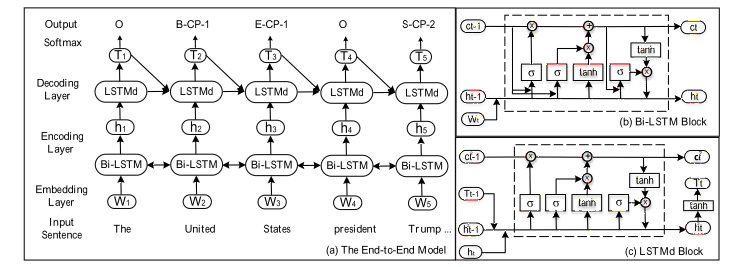• Embedding层

Embedding层将每个词的1-hot表示转换成embedding向量，则词序列可以表示为：

$W={W_1,W_2,W_3,…,W_t,W_{t+1},…,W_n}$,　$W_t$ 表示序列中第t个词汇对应的向量，维度为d,n表示给定句子的长度。

• 双向LSTM编码层

Word embedding层之后是两个平行的的LSTM层，即前向和后向的LSTM层，由一系列循环神经子网组成。每一个时间步长是一个LSTM记忆块。LSTM记忆块从左到右分别是forgate-gate、input-gate和output-gate每一个隐层向量$h_t$ 是由上一时刻的隐层向量$h_{t-1}$ 、细胞状态$c_{t-1}$ 以及当前输入的词向量$W_t$计算得到，如图2(b)所示。计算公式如下: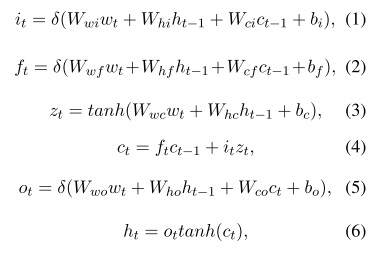其中i,f和o分别是input gate，forget gate和output gate。b是偏置项，c是记忆细胞，W(.)是参数。对于每个单词$\omega_t$ ,前向的LSTM层利用$\omega_1$ 到$\omega_t$ 的上下文信息，得到向量$\overrightarrow {h_t}$ 。类似地，反向的LSTM层利用从$\omega_n$ 到$\omega_t$ 的上下文信息得到向量$\overleftarrow {h_t}$ 。最后，将$\overrightarrow {h_t}$ 和 $\overleftarrow {h_t}$连接来表示单词t编码后的结果$[\overrightarrow {h_t},\overleftarrow {h_t}]$ 。

• LSTM解码层

本文使用LSTM来标注序列,当标注$\omega_t$的标签时，解码层的输入为: Bi-LSTM的输出$h_t$　，前一时刻预测标签对应的embedding$T_{t-1}$ ,前一时刻的记忆细胞值$c_{t-1}^{(2)}$ ,以及解码层前一时刻的输出$h_{t-1}^{(2)}$ ，计算过程如图2(c)所示，计算公式如下: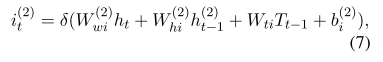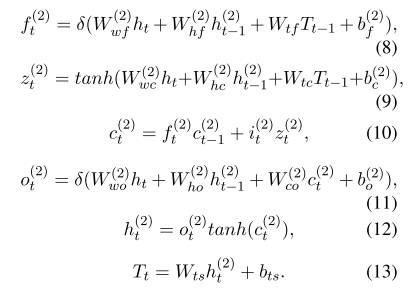最后，通过softmax层计算归一化的实体标签概率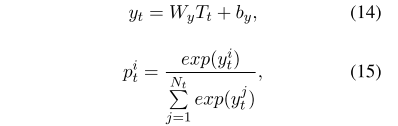### 4 The Bias Objective Function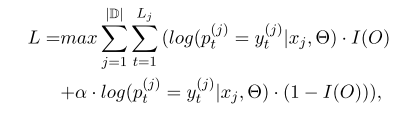$\vert \mathbb D\vert$ 是训练集的大小，$L_j$是句子$x_j$的长度，$y_t^{(j)}$是句子$x_j$中单词t的label，$p_t^{(j)}$ 是正则化的实体标注预测概率值，$I(O)$ 是一个”开关函数”，用来区分tag是否是’O’(即Other)。其表达式如下: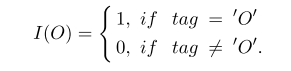$\alpha$是偏置权重，$\alpha$越大，关系标注的结果对模型的影响越大(即实体关系标注对损失函数的影响更大)。

### 5 Experiments

#### Experimental setting

Dataset: 使用NYT(纽约时报)数据集进行验证，该数据集的训练集部分，是由远程监督的方法自动标注的，而测试集部分则是手工进行标注的，训练集包括353k对三元组，测试集包括3880对三元组。关系集合的大小为24。

Evaluation: 采用标准的Precision、Recall和F1-score来评估结果。与传统方法不同的是，本文没有利用实体类型信息来进行三元组提取，因此在评估时不需要考虑实体类型的准确度。当一个三元组的关系类型和两个对应的实体位置都正确时，该三元组被认为是正确的。同时，为了使实验结果更具有说服力，本文将测试数据分为两部分，一部分为全部数据的10%用来得到实验结果，剩下的90%用来验证实验结果的准确性。重复10次实验得到均值和标准差。

**Hyperparameters **: 本文通过word2vec来进行word embedding，word embeddings的维数是300维。编码层的LSTM单元是300个，解码层的LSTM单元数目是600个。Bias Objective Function中的$\alpha$设为10。实验结果如表１所示: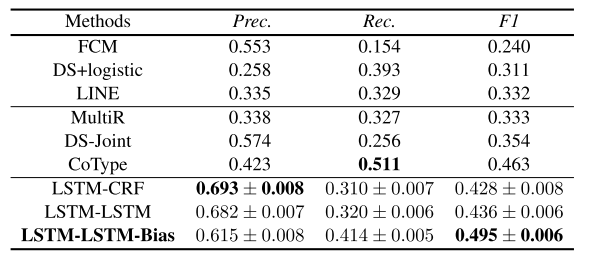Baselines:　Baseline分为３类，一类是将实体抽取和关系抽取当成是两个串行的任务进行，还有一类是其他的联合抽取方法，最后一类是传统的end-to-end标注模型。对比实验的选择详见论文。

### 6 Analysis and Discussion

#### Error Analysis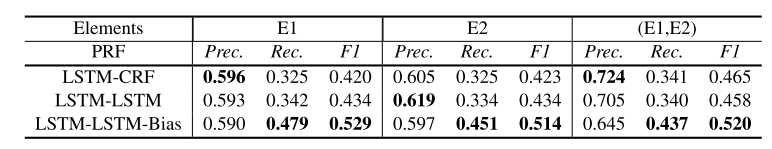#### Analysis of Biased Loss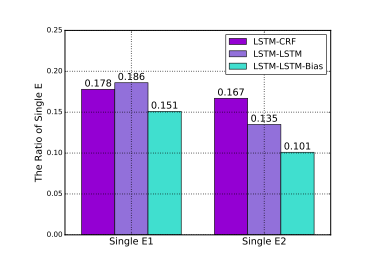Content